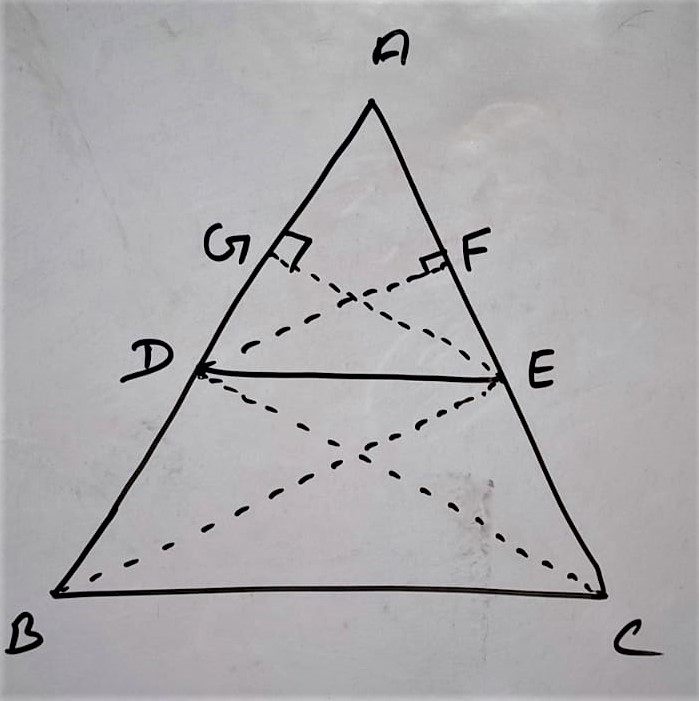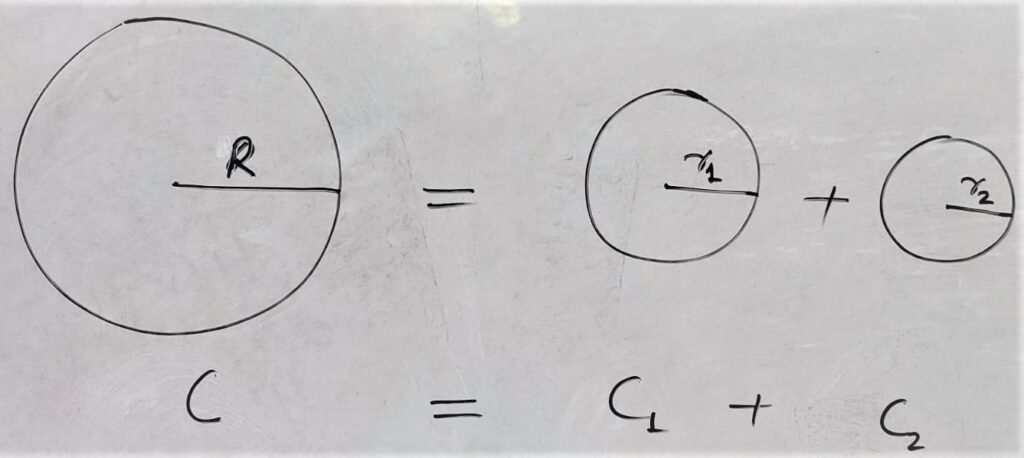# Uncategorized## State and Prove Basic Proportionality (BPT) Theorem Class 10

Theorem 6.1 Thale’s Theorem/ Basic Proportionality Theorem (BPT) Statement: ” If a line is drawn parallel to one side of a triangle to intersect the other two sides in distinct points. The other two sides are divided in the same ratio.” OR Prove that in a triangle a line parallel to one side divides remaining …

## NCERT Trigonometry Class 10 Exercise 8.3 Solutions

Trigonometric Ratios of Complementary Angles  Important identities: 1. sin(90 – θ) = cosθ 2. cos(90-θ) = sinθ 3. tan(90-θ) = cotθ 4. cot(90-θ) = secθ 5. sec(90-θ) = cosecθ 6. cosec(90-θ) = secθ Q.1 Evaluate the following: (i) sin18°/cos72° Solution: sin18°/cos72° = sin( 90°- 72° )/cos72°   [ sin(90-θ) = cosθ ] = cos 72° / …

## NCERT Solution Exercise 13.3 Class 10

Q.1 A metallic sphere of radius 4.2 cm is melted and recast into the shape of a cylinder of radius 6 cm. Find the height of the cylinder. Solution: Volume of cylinder = Volume of metallic sphere ⇒ πr²H       = 4/3 × πr³ ⇒ 6²H         = 4/3 × (4.2)³ ⇒ …

## NCERT Area Related to Circles Class 10 Exercise – 12.3 Solution

Note: In this exercise we will discuss the Areas of Combinations of plane figures . Q.1. Find the area of the shaded region in figure 12.19 , if PQ = 24 cm , PR = 7 cm and O is the center of the circle. Given:  P Q = 24 cm P R = 7 …## NCERT Area Related to Circles Class 10 Chapter 12 Exercise – 12.1 Solution

Important Points: Perimeter of or Circumference of Circle = 2πr Area of Circle = π r² Area of Minor Sector = (θ/360º)  πr² Area of Major Sector = (360º-θ)πr²/360º Length of Arc = (θ/360º) 2 r Area of Minor Segment = [ (θ/360º) π – sin(θ/2)cos(θ/2)] r² Area of Major Segment = Area of Circle …